Main Content

# phased.IsotropicAntennaElement

Isotropic antenna element

## Description

The `phased.IsotropicAntennaElement` object creates an antenna element with an isotropic response pattern. The object models an antenna element whose response is unity in all directions. An isotropic antenna does not support polarization.

To compute the response of the antenna element for specified directions:

1. Create the `phased.IsotropicAntennaElement` object and set its properties.

2. Call the object with arguments, as if it were a function.

To learn more about how System objects work, see What Are System Objects?.

## Creation

### Syntax

``antenna = phased.IsotropicAntennaElement``
``antenna = phased.IsotropicAntennaElement(Name,Value)``

### Description

example

````antenna = phased.IsotropicAntennaElement` creates an isotropic antenna System object™, `antenna`, with default property values.`antenna = phased.IsotropicAntennaElement(Name,Value)` creates an isotropic antenna object, `antenna`, with each specified property `Name` set to the specified `Value`. You can specify additional name-value pair arguments in any order as (`Name1`,`Value1`,...,`NameN`,`ValueN`).```

## Properties

expand all

Unless otherwise indicated, properties are nontunable, which means you cannot change their values after calling the object. Objects lock when you call them, and the `release` function unlocks them.

If a property is tunable, you can change its value at any time.

For more information on changing property values, see System Design in MATLAB Using System Objects.

Operating frequency range of the antenna, specified as a nonnegative, real-valued, 1-by-2 row vector in the form `[LowerBound HigherBound]`. The antenna element has no response outside the specified frequency range. Units are in Hz.

Data Types: `double`

Backbaffle the antenna element, specified as `false` or `true`. Set this property to `true` to baffle the response on the backside of the antenna element. In this case, the antenna response to all azimuth angles beyond ±90° from broadside (0° azimuth and 0°elevation) is zero. When the value of this property is `false`, the back of the antenna element is not baffled.

Data Types: `logical`

## Usage

### Syntax

``RESP = antenna(FREQ,ANG)``

### Description

example

````RESP = antenna(FREQ,ANG)` returns the antenna voltage response `RESP` at operating frequencies specified in `FREQ` and in directions specified in `ANG`.```

### Input Arguments

expand all

Operating frequency of antenna element, specified as a nonnegative scalar or nonnegative, real-valued, 1-by-L row vector. Frequency units are in Hz.

`FREQ` must lie within the range of values specified by the `FrequencyRange` or the `FrequencyVector` property of the element. Otherwise, the element produces no response and the response is returned as `–Inf`. Most elements objects use the `FrequencyRange` property except for `phased.CustomAntennaElement`, which uses the `FrequencyVector` property.

Example: `[1e8 2e6]`

Data Types: `double`

Azimuth and elevation angles of response directions, specified as a real-valued, 1-by-M row vector or a real-valued, 2-by-M matrix, where M is the number of angular directions. Angle units are in degrees. The azimuth angle must lie in the range –180° to 180°, inclusive. The elevation angle must lie in the range –90° to 90°, inclusive.

• If `ANG` is a 1-by-M vector, each element specifies the azimuth angle of the direction. In this case, the corresponding elevation angle is assumed to be zero.

• If `ANG` is a 2-by-M matrix, each column of the matrix specifies the direction in the form [azimuth; elevation].

The azimuth angle is the angle between the x-axis and the projection of the direction vector onto the xy plane. This angle is positive when measured from the x-axis toward the y-axis. The elevation angle is the angle between the direction vector and xy-plane. This angle is positive when measured towards the z-axis. See the definition of Azimuth and Elevation Angles.

Example: `[110 125; 15 10]`

Data Types: `double`

### Output Arguments

expand all

Voltage response of antenna element, returned as a complex-valued M-by-L matrix. In this matrix, M represents the number of angles specified in `ANG` and L represents the number of frequencies specified in `FREQ`.

Data Types: `double`

## Object Functions

To use an object function, specify the System object as the first input argument. For example, to release system resources of a System object named `obj`, use this syntax:

`release(obj)`

expand all

 `beamwidth` Compute and display beamwidth of sensor element pattern `directivity` Directivity of antenna or transducer element `isPolarizationCapable` Antenna element polarization capability `pattern` Plot antenna or transducer element directivity and patterns `patternAzimuth` Plot antenna or transducer element directivity and pattern versus azimuth `patternElevation` Plot antenna or transducer element directivity and pattern versus elevation
 `step` Run System object algorithm `release` Release resources and allow changes to System object property values and input characteristics `reset` Reset internal states of System object

## Examples

collapse all

Create an isotropic antenna operating over a frequency range from 800 MHz to 1.2 GHz. The operating frequency is 1 GHz. Find the response of the antenna at boresight. Then, plot the polar-pattern elevation response of the antenna.

```antenna = phased.IsotropicAntennaElement( ... 'FrequencyRange',[800e6 1.2e9]); fc = 1e9;```

Obtain the response at boresight.

`resp = antenna(fc,[0;0])`
```resp = 1 ```

Plot the response pattern.

```pattern(antenna,fc,0,[-90:90],'CoordinateSystem','polar', ... 'Type','powerdb','Normalize',true)```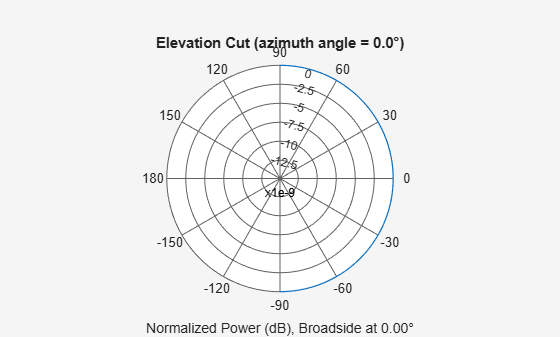Create an isotropic antenna element using the `phased.IsotropicAntennaElement` System object™ and show that it does not support polarization.

```antenna = phased.IsotropicAntennaElement('FrequencyRange',[1.0,10]*1e9); isPolarizationCapable(antenna)```
```ans = logical 0 ```

The returned value `0` shows that the antenna element does not support polarization.

Compute the directivity of an isotropic antenna element in different directions.

Create an isotropic antenna element system object.

`antenna = phased.IsotropicAntennaElement();`

First, specify that the directions of interest all at an elevation angle of zero degrees. The seven azimuth angles are centered around boresight (zero degrees azimuth and zero degrees elevation). Set the frequency to 1 GHz.

```ang = [-30,-20,-10,0,10,20,30; 0,0,0,0,0,0,0]; freq = 1e9;```

Compute the directivity along the constant elevation cut.

`d = directivity(antenna,freq,ang)`
```d = 7×1 0 0 0 0 0 0 0 ```

Next, specify that the directions of interest all at an azimuth angle of zero degrees. All elevation angles are centered around boresight. The five elevation angles range from $-20$ to $+20$ degrees, inclusive. Set the frequency to 1 GHz.

```ang = [0,0,0,0,0; -20,-10,0,10,20]; freq = 1e9;```

Compute the directivity along the constant azimuth cut.

`d = directivity(antenna,freq,ang)`
```d = 5×1 0 0 0 0 0 ```

For an isotropic antenna, the directivity is independent of direction.

Create an isotropic antenna element. Then, plot the antenna power pattern and directivity.

First, create the antenna.

`antenna = phased.IsotropicAntennaElement;`

Draw an azimuth cut of the power pattern at 0 degrees elevation. Assume the operating frequency is 1 GHz.

```fc = 1e9; pattern(antenna,fc,[-180:180],0,... 'Type','power',... 'CoordinateSystem','rectangular')```Draw the same azimuth cut of the antenna directivity.

```pattern(antenna,fc,[-180:180],0,... 'Type','directivity',... 'CoordinateSystem','rectangular')```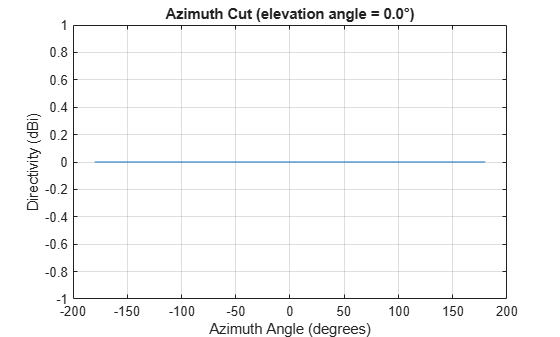Construct an isotropic antenna operating in the frequency range from 800 MHz to 1.2 GHz. Compute the response at boresight at 1 GHz. Display the power pattern of the antenna at 1 GHz.

```antenna = phased.IsotropicAntennaElement(... 'FrequencyRange',[800e6 1.2e9]); fc = 1e9; resp = antenna(fc,[0;0])```
```resp = 1 ```

Plot the elevation power pattern of the antenna in polar coordinates.

`pattern(antenna,fc,0,[-90:90],'Type','powerdb','CoordinateSystem','polar')`Construct an isotropic antenna operating over a frequency range from 800 MHz to 1.2 GHz. Then, plot the 3-D antenna field pattern.

Construct an isotropic antenna element.

```antenna = phased.IsotropicAntennaElement(... 'FrequencyRange',[800e6 1.2e9]);```

Plot the 3-D magnitude pattern of the antenna at 1 GHz from $-30$ to $30$ degrees in both azimuth and elevation in 0.1 degree increments.

```fc = 1e9; pattern(antenna,fc,[-30:0.1:30],[-30:0.1:30],... 'Type','efield',... 'CoordinateSystem','polar')```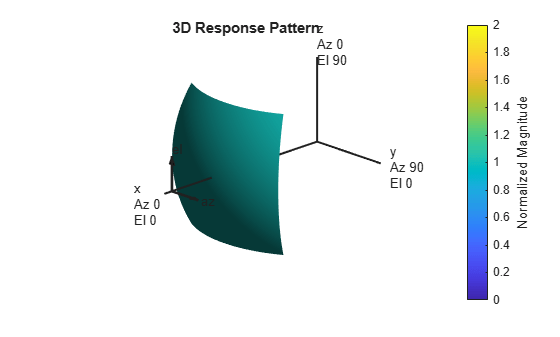Plot an azimuth cut of the directivity of an isotropic antenna element at 0 degrees elevation for all azimuth angles and at 30 degrees elevation for a small span of azimuth angles. Assume the operating frequency is 500 MHz.

Create the antenna element.

```fc = 500e6; antenna = phased.IsotropicAntennaElement('FrequencyRange',[100,900]*1e6);```

Plot the pattern for all azimuth angles at 0 degrees elevation.

`patternAzimuth(antenna,fc,0)`Plot the pattern for a reduced span of azimuth angles using the `Azimuth` parameter.

`patternAzimuth(antenna,fc,30,'Azimuth',[-20:20])`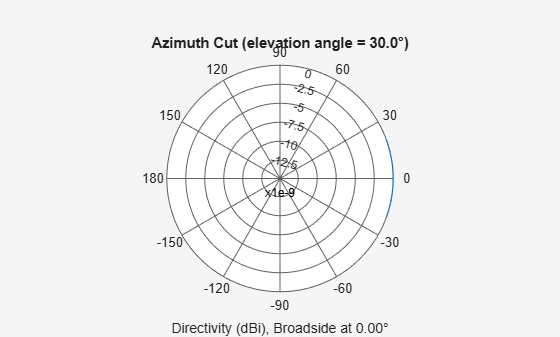Plot an elevation cut of directivity of an isotropic antenna element at 45 degrees azimuth for all elevation angles and at 45 degrees for a span of elevation angles. Assume the operating frequency is 500 MHz.

Create the antenna element.

```fc = 500e6; antenna = phased.IsotropicAntennaElement('FrequencyRange',[100,900]*1e6);```

Plot the directivity for all elevation angles.

`patternElevation(antenna,fc,45)`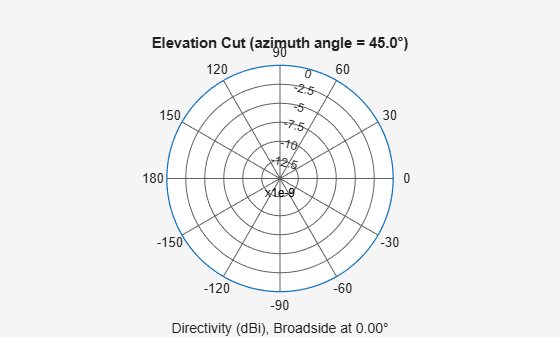Plot the directivity for a span of elevation angles using the `Elevation` parameter.

`patternElevation(antenna,fc,45,'Elevation',[-20:20])`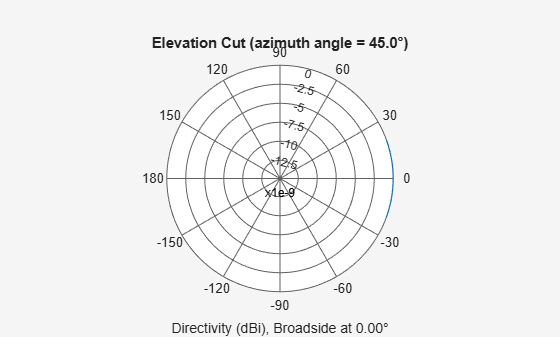## Extended Capabilities

Introduced in R2011a

## Support

#### Exploring Hybrid Beamforming Architectures for 5G Systems

Download white paper